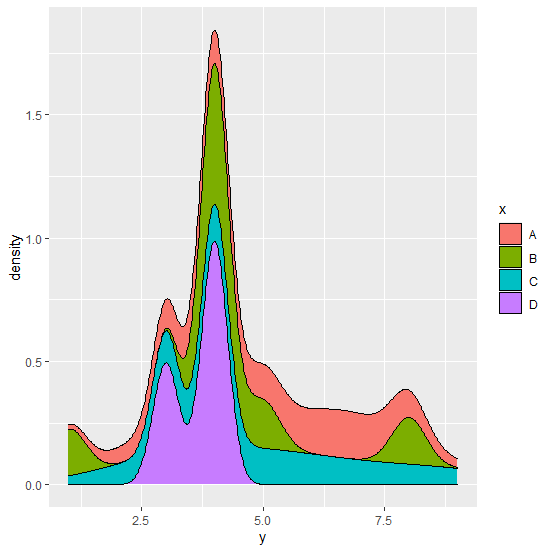# How to create stacked plot with density using ggplot2 in R?

To create stacked plot with density using ggplot2, we can use geom_density function of ggplot2 package and position="stack". For example, if we have a data frame called df that contains two columns say x and y, where x is categorical and y is numerical then the stacked plot with density can be created by using the command −

ggplot(df,aes(y,y=..density..))+geom_density(aes(fill=x),position="stack")

## Example

Consider the below data frame −

Live Demo

> x<-sample(LETTERS[1:4],20,replace=TRUE)
> y<-rpois(20,5)
> df<-data.frame(x,y)
> df

## Output

   x y
1  C 3
2  C 5
3  B 4
4  A 7
5  B 1
6  A 6
7  D 4
8  C 3
9  C 7
10 B 4
11 D 3
12 C 9
13 A 4
14 A 3
15 B 4
16 B 8
17 A 7
18 C 5
19 D 4
20 B 5

Loading ggplot2 package and creating stacked plot with density of y −

> library(ggplot2)
> ggplot(df,aes(y,y=..density..))+geom_density(aes(fill=x),position="stack")

## Output